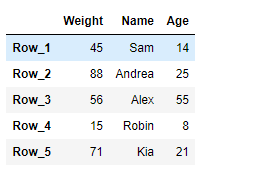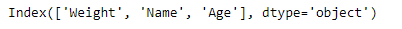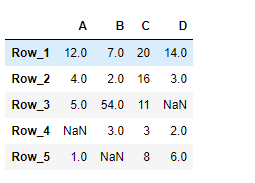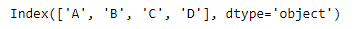# Python | Pandas DataFrame.columns

• Last Updated : 20 Feb, 2019

Pandas DataFrame is a two-dimensional size-mutable, potentially heterogeneous tabular data structure with labeled axes (rows and columns). Arithmetic operations align on both row and column labels. It can be thought of as a dict-like container for Series objects. This is the primary data structure of the Pandas.

Pandas` DataFrame.columns` attribute return the column labels of the given Dataframe.

Syntax: DataFrame.columns

Parameter : None

Returns : column names

Example #1: Use `DataFrame.columns` attribute to return the column labels of the given Dataframe.

 `# importing pandas as pd``import` `pandas as pd`` ` `# Creating the DataFrame``df ``=` `pd.DataFrame({``'Weight'``:[``45``, ``88``, ``56``, ``15``, ``71``],``                   ``'Name'``:[``'Sam'``, ``'Andrea'``, ``'Alex'``, ``'Robin'``, ``'Kia'``],``                   ``'Age'``:[``14``, ``25``, ``55``, ``8``, ``21``]})`` ` `# Create the index``index_ ``=` `[``'Row_1'``, ``'Row_2'``, ``'Row_3'``, ``'Row_4'``, ``'Row_5'``]`` ` `# Set the index``df.index ``=` `index_`` ` `# Print the DataFrame``print``(df)`

Output :Now we will use `DataFrame.columns` attribute to return the column labels of the given dataframe.

 `# return the column labels``result ``=` `df.columns`` ` `# Print the result``print``(result)`

Output :As we can see in the output, the `DataFrame.columns` attribute has successfully returned all of the column labels of the given dataframe.

Example #2: Use `DataFrame.columns` attribute to return the column labels of the given Dataframe.

 `# importing pandas as pd``import` `pandas as pd`` ` `# Creating the DataFrame``df ``=` `pd.DataFrame({``"A"``:[``12``, ``4``, ``5``, ``None``, ``1``], ``                   ``"B"``:[``7``, ``2``, ``54``, ``3``, ``None``], ``                   ``"C"``:[``20``, ``16``, ``11``, ``3``, ``8``], ``                   ``"D"``:[``14``, ``3``, ``None``, ``2``, ``6``]}) `` ` `# Create the index``index_ ``=` `[``'Row_1'``, ``'Row_2'``, ``'Row_3'``, ``'Row_4'``, ``'Row_5'``]`` ` `# Set the index``df.index ``=` `index_`` ` `# Print the DataFrame``print``(df)`

Output :Now we will use `DataFrame.columns` attribute to return the column labels of the given dataframe.

 `# return the column labels``result ``=` `df.columns`` ` `# Print the result``print``(result)`

Output :As we can see in the output, the `DataFrame.columns` attribute has successfully returned all of the column labels of the given dataframe.

My Personal Notes arrow_drop_up